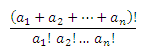## Basic math functions: gcd, lcm, mround, multinomial, sqrt, sqrtpi

#### Excel Function GCD

The GCD() function is used to determine the greatest common divisor of a group of numbers.

This function can take more than two arguments but are limited to a maximum of 255.

The Syntax is : GCD(num1,num2�num255)

 GCD() Remarks Formula 1 NA =GCD(2,5,4,3) 2 NA =GCD(2,4,6,8,10) #NUM! Contains negative number =GCD(-2,4,7,90) 2 NA =GCD(0.1,2,0.8) 2 NA =GCD(200,102)

#### Excel Function LCM

The LCM() function is used to determine the lowest common multiple of a given set of numbers.

This function too is limited to a maximum of 255 arguments.

The Syntax is LCM(num1,num2,..num255)

 LCM() Remarks Formula 60 NA =LCM(2,5,4,3) 120 NA =LCM(2,4,6,8,10) #NUM! Contains negative number =LCM(-2,4,7,90) 0 NA =LCM(0.1,2,0.8) 10200 NA =LCM(200,102)

#### Excel Function MROUND

The MROUND() function rounds the specified number to the nearest multiple of the given number.

This function rounds the number either up or down whichever is nearer.

This function takes two arguments.

The Syntax is : MROUND(number, multiple)

 Number Multiple MROUND() Formula 2 5 0 =MROUND(L29,M29) 3 2 4 =MROUND(L30,M30) 5 8 8 =MROUND(L31,M31) 7 -5 #NUM! =MROUND(L32,M32) -9 13 #NUM! =MROUND(L33,M33)

As seen from the above, a negative number on either of the arguments results in an error.

#### Excel Function MULTINOMIAL

The MULTINOMIAL() function calculates the multinomial of the supplied numbers.

A multinomial is nothing but the ratio of factorial of sum of the values to the factorial of its products.

The formula is as given:This function can take a maximum of 255 arguments.

 MULTINOMIAL() Remarks Formula 105 NA =MULTINOMIAL(1,2,4) #NUM! Negative number =MULTINOMIAL(1,-2,4) 5 NA =MULTINOMIAL(1,0.2,4)

#### Excel Function SQRT

The SQRT() function is used to calculate the square root of a given number.

The important thing is that, this function calculates the square root of positive numbers only.

The syntax is SQRT(number)

 SQRT() Remarks Formula 10 No error =SQRT(100) #NUM! Negative number =SQRT(-4) #NAME? Non numeric values =SQRT(a)

#### Excel Function SQRTPI

The SQRTPI()Function returns the square root of the product of a given number and the constant pi.

The mathematical constant pi=3.14.

There is no need to explicitly multiply the value by pi as it will be done automatically.

The Syntax is SQRTPI(number).

 SQRTPI() Remarks Formula 17.7245385090552 No error =SQRTPI(100) #NUM! Negative number =SQRTPI(-4) #NAME? Non numeric values =SQRTPI(a)